Select Page

# Averages

## Introduction

We have already learned that it is difficult to learn anything from the raw unless and until the data is arranged in proper manner. When the data have been arranged into a frequency distribution, the information contained in the data could be easily understood. Now we will move a step ahead and find a single value, which will represent all the values of the distribution in some particular way. A value which is being used in this way to represent the distribution is called an average. Since the averages tend to lie in the center of the distribution, they are called measures of central tendency.

### Types of Averages

The most commonly used average are;

i. Mean

a. Arithmetic Mean

b. Geometric Mean

c. Harmonic Mean

ii. Median

iii. Mode

### Qualities of a Good Average:

i. It should be properly defined, preferably by a mathematical formula, so that different individuals working with the same data should get the same answer unless there are mistakes in calculations.

ii. It should be simple to understand and easy to calculate.

iii. It should be based on all the observations so that if we change the value of any observation, the value of the average should also be changed.

iv. It should not be unduly affected by extremely large or extremely small values.

v. It should be capable of algebraic manipulation. By this we mean that if we are given the average heights for different groups, then the average should be such that we can find the combined average of all groups taken together.

vi. It should have quality of sampling stability. That is, it should not be affected by the fluctuations of sampling. For example, if we take ten or twelve samples of twenty students easch and find the average height for each sample, we should get approximately the same average height for each sample.

## Mean

### Introduction

Mean is one of the types of averages. Mean is further divided into three kinds, which are the arithmetic mean, the geometric mean and the harmonic mean. These kinds are explained as follows;

## Arithmetic Mean:

The arithmetic mean is most commonly used average. It is generally referred as the average or simply mean. The arithmetic mean or simply mean is defined as the value obtained by dividing the sum of values by their number or quantity. It is denoted as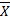(read as X-bar). Therefore, the mean for the values X1, X2, X3,……….., Xn shall be denoted by. Following is the mathematical representation for the formula for the arithmetic mean or simply, the mean.

For Example:

i. The arithmetic mean of the values 5, 8, 10, 12 and 17 is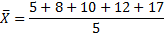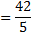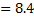ii. Total annual incomes of eight families are Rs.3200, Rs.3500, Rs.4500, Rs.3800, Rs.4200, Rs.3600, and Rs.53200. Their arithmetic mean is obtained as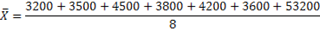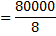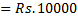iii. The mean wage of 5 employees is Rs.1000. If the wages of four employees are Rs.800, Rs.1200, Rs.1300 and Rs.900, find the wage of fifth employee.

Sum of wages of 5 employees: ∑X = n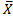= 5(1000) = Rs.5000

Sum of wages of 4 employees = 800 + 1200 + 13000 + 900 = Rs.4200

Wages of the fifth employees = 5000 – 4200 = Rs.800.

iv. For the set 15 observations, the mean came out to be 18.2. Later on checking it was discovered that an observation 19.7 was incorrectly recorded whereas the correct value was 17.9. Calculate the correct mean from this information.

Or, ∑X = n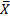= 15(18.2) = 271.2

∑X (corrected) = ∑X + correct value – incorrect value

= 273.0 + 17.9 – 19.7

= 271.2

### Arithmetic Mean for Grouped Data:

The formula provided above is being used when the number of values is small. If the number of values is large, they are grouped into a frequency distribution. In case of grouped data when the data is arranged in the form of frequency distribution, all the values falling in a class are assumed to be equal to the class mark or midpoint. If the X1, X2, ……, Xk are the class marks with f1, f2, ….., fk as the corresponding class frequencies, the sum of the values in the first class would be f1X1, in the second class f2X2 and so on the sum of the values in kth class would be fkXk. Hence, the sum of the values in all the k classes would be

f1X1 + f2X2 + ……. + fkXk = ∑fX

The total number of values is the sum of the class frequencies, as follows;

f1 + f2 + ……… + fk = ∑f

Since, the mean is the value obtained by dividing the sum of the values by their number, the mean for grouped data is calculated by the following formula;

For Example:

Find the mean weight of 120 weight students at a university from the following frequency distribution Table 11

Table 11
 Weight (lb) Class Mark (X) Frequency (f) fX 110 – 119 114.5 1 114.5 120 – 129 124.5 4 498 130 – 139 134.5 17 2286.5 140 – 149 144.5 28 4046 150 – 159 154.5 25 3862.5 160 – 169 164.5 18 2961 170 – 179 174.5 13 2268.5 180 – 189 184.5 6 1107 190 – 199 194.5 5 972.5 200 – 209 204.5 2 409 210 -219 214.5 1 214.5 n = ∑f = 120 ∑fX = 18740

From the above table we concluded that n = ∑f = 120 and ∑fX = 18740, by using the formula of arithmetic mean for grouped data the mean weight will be;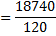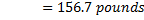### Weighted Arithmetic Mean:

When the values are not of equal importance, we assign them certain numerical values to express their relative importance. These numerical values are called weights. If X1, X2, ……, Xk have weights W1, W2, ……., W3, then the weighted arithmetic mean or the weighted mean, which is denoted as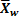, is calculated by the following formula;

Thus the mean of grouped data may be regarded as the weighted mean of the values of the values X1, X2, ……, Xk whose weights are the respective class frequencies f1, f2, ….., fk.

For Example:

The marks obtained by a student in English, Urdu and Statistics were 70, 76, and 82 respectively. Find the appropriate average if weights of 5, 4 and 3 are assigned to these subjects.

We will use the weighted mean, the weights attached to the marks being 5, 4 and 3. Thus,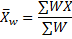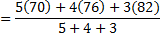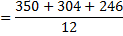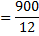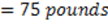## Geometric Mean:

The geometric mean, G, of a set of n positive values X1, X2, ……, Xn is the nth root of the product of the values. Mathematically the formula for geometric mean will be as follows;

For Example:

The geometric mean of the values 2, 4 and 8 will be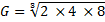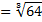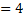In practice, it is difficult to extract higher roots. The geometric mean is, therefore, computed using logarithms. Mathematically, it will be represented as follows;

Here we assume that all the values are positive, otherwise the logarithms will be not defined.

For Example:

Find the geometric mean for the values 3, 5, 6, 6, 7, 10, 12.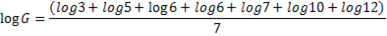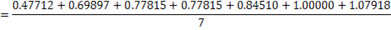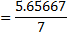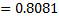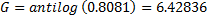The arithmetic mean of the above values will be,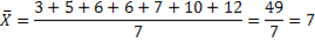This shows that the geometric men of the set of values, not all equal, are less than their arithmetic mean. Moreover, if any one of the original values is zero, their geometric mean will be zero, if log formula used for calculating geometric mean.

### Geometric Mean for Grouped Data:

When the data have been arranged into a frequency distribution, each of the original observation in a class is assumed to have a value equal to its class marks. Suppose X1, X2, ……, Xk represents the class marks in a frequency distribution with f1, f2, ….., fk as the corresponding class frequencies, where f1 + f2 + ……… + fk = ∑f = n. since X1 occurs f1 times, X2 occurs f2 times,………., Xk occurs fk times, then the formula for the geometric mean will be as;

Where, n = ∑f. This is sometimes called the weighted geometric mean with weights f1, f2, ….., fk. In case of using logarithm, the above formula will become as;

For Example:

i. The geometric mean for the following distribution tables, by using the basic formula is as follows;
 X f 1 2 2 3 3 4 4 1 Total 10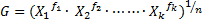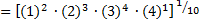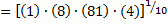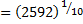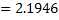ii. The geometric mean for the following frequency distribution table by using the logarithm formula is as follows;

 X f log X f log X 1 2 0 0 2 3 0.301029996 0.903089987 3 4 0.477121255 1.908485019 4 1 0.602059991 0.602059991 ∑f = 10 ∑f log X = 3.4135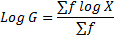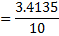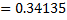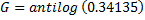iii. The geometric mean of the weights of 120 students at a university will be calculated by using the following Table 12

Table 12

 Weight (lb) Class Mark (X) Frequency (f) log X f log X 110 – 119 114.5 1 2.058805487 2.058805487 120 – 129 124.5 4 2.095169351 8.380677406 130 – 139 134.5 17 2.128722284 36.18827883 140 – 149 144.5 28 2.159867847 60.47629972 150 – 159 154.5 25 2.188928484 54.72321209 160 – 169 164.5 18 2.216165902 39.89098624 170 – 179 174.5 13 2.241795431 29.14334061 180 – 189 184.5 6 2.26599637 13.59597822 190 – 199 194.5 5 2.288919606 11.44459803 200 – 209 204.5 2 2.310693312 4.621386625 210 -219 214.5 1 2.331427297 2.331427297 n = ∑f = 120 ∑f log X = 262.8549906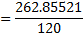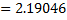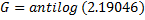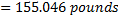## Harmonic Mean:

The harmonic mean, H, of a set of n values X1, X2, ……, Xn is the reciprocal of the arithmetic mean of the reciprocals of the values. Mathematically, the formula for harmonic mean will be as follows;

For Example:

The harmonic mean of the value 3, 5, 6, 6, 7, 10 and 12 will be as follows;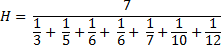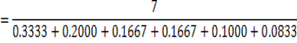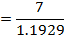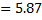### Harmonic Mean for Grouped Data:

Suppose X1, X2, ……, Xk represents the class marks in a frequency distribution with f1, f2, ….., fk as the corresponding class frequencies, where f1 + f2 + ……… + fk = ∑f = n. Then the reciprocals of the class marks will be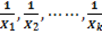. Since, the reciprocals occur with frequencies f1 + f2 + ……… + fk, the total value of the reciprocals in the first class is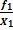, in the second class is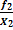, ……., in the kth class is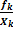. The formula for calculating harmonic mean for grouped data will be as follows;

Where, n = ∑f. This is sometimes called the weighted geometric mean with weights f1, f2, ….., fk..

For Example:

The harmonic mean of the frequency distribution of weights of 120 students at a university, is calculated by using the following Table 13

Table 13

 Weight (lb) Class Mark (X) Frequency (f)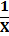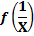110 – 119 114.5 1 0.008734 0.008734 120 – 129 124.5 4 0.008032 0.032129 130 – 139 134.5 17 0.007435 0.126394 140 – 149 144.5 28 0.00692 0.193772 150 – 159 154.5 25 0.006472 0.161812 160 – 169 164.5 18 0.006079 0.109422 170 – 179 174.5 13 0.005731 0.074499 180 – 189 184.5 6 0.00542 0.03252 190 – 199 194.5 5 0.005141 0.025707 200 – 209 204.5 2 0.00489 0.00978 210 -219 214.5 1 0.004662 0.004662 ∑f = n = 120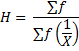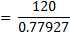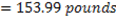The author is currently doing Chartered Accountancy in Pakistan and has qualified c.a inter. She is Graduate in Bachelors in Commerce from Punjab University, Lahore. And has extensive knowledge in Statistics, Economics, Accounting and Auditing. She has done internship at Ernst & Young, Ford, Rhodes, Sidat Hyder in Advisory department as an Internal Auditor. She has worked for SEO Consultant as SEO Content Writer for more than a year. She is currently a contributing author of Econtutorials.com.
Shares